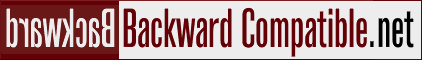# Performance of PHP if, switch, arrays... using "B-tree-if" as solution

For the game I'm making I have a bunch of array which represent a puzzle player needs to solve. Ensuring puzzle is solvable is CPU intensive, so I pre-calculated a couple of thousands of puzzles and select one randomly. Since puzzles are static data which is not going to change, I decided not to burden the database with this because DBMS is always overloaded with other stuff anyway.

My first thought was to build a big array and fetch a random element. I found some benchmark showing that this is faster than "if" or "switch", however benchmarks excluded time needed to parse/create the array itself. Since every player is a new HTTP request, this huge array would have to be constructed each time. I am using APC, but I failed to find if arrays in precompiled PHP source file are stored "structured" as well.

Dropping the array idea, I though about "switch", foolishly thinking that it would use some kind of jump-table and run the desired return statement. Something like this:

```function get_puzzle(\$id)
{
switch (\$id)
{
case 0: return array (...first puzzle...);
case 1: return array (...second puzzle...);
case 2: return array (...etc.
```

However, researching this I find out that switch performs similar to series of "if" statements... variable is compared with each "case".

So I decided to roll my own solution using "if" statements, but not linear. I used a B-tree approach. Split by two until only one is left. This means it would take only 11 comparisons to reach a puzzle from a set of 2048. Here's an example with set of 256 puzzles.

```function get_puzzle_btree(\$id)
{
if (\$id < 128)
if (\$id < 64)
if (\$id < 32)
if (\$id < 16)
if (\$id < 8)
if (\$id < 4)
if (\$id < 2)
if (\$id < 1)
return array (...first puzzle...);
else
return array (...second puzzle...);
...etc.```

Of course, I did not write this "if" behemoth by hand. A simple 20-line recursive function spits out the PHP code.

In the end, I wrote a simple comparison loop that tries to get all the puzzles and compares whether the old "switch" and new "btree" function return the same values.

Milan Babuškov, 2011-12-01